﻿ A Heterogeneous Bulk Service Queueing Model With VacationPublications are Open
Access in this journal
Article Versions
Export Article
• Normal Style
• MLA Style
• APA Style
• Chicago Style
Research Article
Open Access Peer-reviewed

### A Heterogeneous Bulk Service Queueing Model With Vacation

R. Sree Parimala
Journal of Mathematical Sciences and Applications. 2020, 8(1), 1-5. DOI: 10.12691/jmsa-8-1-1
Received March 09, 2020; Revised April 18, 2020; Accepted May 06, 2020

### Abstract

This paper is concerned with the study of bulk service M/M(a,b)/(2,1) queueing system of two heterogeneous servers with different service rates. In this model it is assumed that the arrival pattern is Poisson style with parameter λ and the service times are assumed to be mutually independent and exponentially distributed with parameters μ1and μ2 for the fast and slow servers respectively. The arrivals are served in batches according to FCFS discipline. In this model, the fast server (server 1) is always retained in the system and a delayed and single vacation policy for slow server (server 2)is discussed. The steady state solutions and the system characteristics are derived and analyzed for this model. The analytical results are numerically exemplified for different values of the parameters and levels also.

### 1. Introduction

The queuing theory is a useful statistical technique for solving peculiar problems. The last two decades have perceived a marvelous growth in the applications of bulk queues to many cramming situations. The primary reason for this is that bulk queueing models are often encountered in real life systems such as shipping systems, computer system, telecommunication, airline arrangement as well as industrial progressions such as production/ inventory systems etc.

Queueing theory is a set of mathematical tools for the analysis of probabilistic systems of customers and servers. It is also known as the theory of overloading, the branch of operational research that discovers the relationship between demand on a service system and the delays grieved by the users of that system. It is measured by one of the standard methodologies with linear programming, simulation and management science because the outcomes are often used when making business judgments about the resources needed to deliver service.

The considerable attention was paid to analyze the queueing system with vacation. Many researchers have analyzed vacation queueing problems for both Markovian and Non-Markovian queueing models. The Markovian models may be more appropriate in practice and have certain advantages. For a detailed comprehensive survey on queueing systems with server vacations and priority systems, one can refer to 3, 4, 5, 7. The study of bulk queues was originated by 6. 2 and 5 are highlighted the applications of bulk queues. The queueing systems with bulk arrival or bulk service or both bulk arrival and bulk service have been extensively studied by many authors. Its wide range of practical applications in a variety of situations makes it attractive to many researchers to work on various queueing models and to obtain closed form solutions.

7 and 8 considers that units are served in batches of not more than b (say). If, immediately after the completion of a service, the server finds more than b units waiting, he takes a batch of b units for service while others wait; if he finds n units (0 ≤ n ≤ b), he takes all the n units in a batch for service. 3 Considered the same rule with the restriction that n ≠ 0 (1≤ n ≤b), that is the service facility stops until a unit arrives. This rule will be called a usual bulk service rule, while Bailey; rule will be its modified type called as bulk service rule with intermittently available server and points out that the distribution of the queue length for the modified rule can be obtained from that of the usual rule.

The rule with a fixed batch size n has been considered by 9 and others. In this case the server waits until there are n units, and then serves all the n units in a batch. If there are more than n units waiting when the server becomes free, he takes a batch of n for service, while others wait. 11 considers this rule: if immediately after the completion of a service, the server finds less than ‘a’ units present, he waits until there are ‘a’ units, whereupon he takes the batch of a units for service; if he finds a or more but atmost b, he takes them all in the batch and if he finds more than b, he takes in the batch for service b units, while others wait. The batch takes a minimum of a units and a maximum of b units. This rule will be called general bulk service rule.

10 Introduced the concept of heterogeneity in service. He discussed the situation of certain hyper exponential distributions of service times with parallel channels. 12 Further discussed more problems by assigning the different service ratesand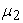to the two branches respectively. 13 Extended this problem by assigning customers to the two servers with probabilities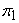andrespectively.

9 have discussed the model M/M(a,b)/2 with heterogeneous servers and obtained the steady state probabilities and busy period distributions. 12 has studied the same model with balking. A bulk queueing model M/M(a,b)/(2,1) for non-identical servers with vacation is studied by 13. In the literature described above, customer inter-arrival times and customer service times are required to follow certain probability distributions with fixed parameters.

The present investigation, an attempt has been made to analyze two heterogeneous M/M(a,b)/(2,1) queueing system with different service rate in which the fast server is always available in the system for service and the slow server can avail the vacation if there are less than ‘a-1’ customers in the queue. That is, delayed vacation for slow server id discussed. The study of this queueing model is organized as follows. The model is described in Section 2. Queueing model is formulated mathematically along with notations in Section 3. The steady state solutions have been obtained in Section 4. The performance measures and mean queue length are derived in Section 5. The numerical results and graphical illustrations are discussed to facilitate the sensitivity analysis in Section 6 and 7. Concluding remarks and notable features of investigation done are highlighted in Section 8.

### 2. Problem Description

In this model it is assumed that the arrival pattern is Poisson with parameter λ. Service is done in batches according to the general bulk service rule introduced by Neuts (1967). The late arrivals are not allowed to join the ongoing service. The successive service times are assumed to be mutually independent and exponentially distributed with parameters μi (i = 1, 2) for each of the two servers (fast and slow) and>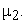On completing the service if the slow server finds less than ‘a’ or ‘a – 1’ customers in the queue and the fast server is busy, he wait in the system for a random period of time before going for vacation, which is called delayed vacation which is exponentially distributed with parameter θ and on returning from vacation, if the slow server finds less than ‘a’ waiting customers and the fast server is busy or idle in the system, he stay in the system until he finds at least ‘a’ customers. i.e., in this system single vacation for slow server is also considered.

The model leads to the state space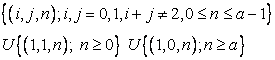In the state (i, j, n), the index i=0 refers to the situation that the fast server is idle and i=1 means that he is busy and the index j=0 refers to the state that the slow server is on vacation and j=1 that he is busy. The index n ≥ 0 refers to the number of waiting customers in the queue.

Defining pi,j,n(t) as the probability that the system is in the state (i, j, n), i, j = 0,1 and n ≥ 0 and assuming that the steady state probabilities exists, the balance equation in the steady state are given by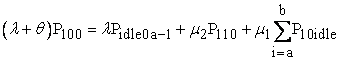(1)(2)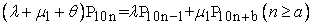(3)(4)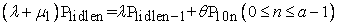(5)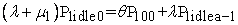(6)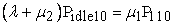(7)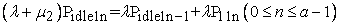(8)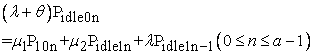(9)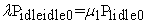(10)

### 4. Computation of Steady State Solutions

Let E denote the forward shifting operator defined by E(P10n) = P10n+1, from equation (3) implies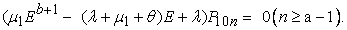The characteristic equation of has only one real root inside the circle |Z| =1 by Rouche’s theorem whenis less than 1. If r0 (say) is the root of the above characteristic equation with |r0|<1,

Then p10n= A1r0n, (n ≥ a), is the solution for the homogeneous difference equation (3), we have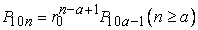(11)

Using equation (2), we get,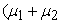) Eb+1E +)= -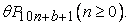The characteristic equation of this equation has only one real root r1 by Rouche’s theorem which lies in the interval (0,1),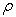< 1, where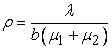and after simplification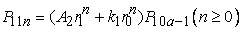(12)

Where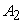is a constant and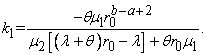From equation (5),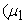Eb+1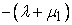E +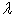)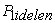= -0 for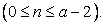The above equation has only one real root r2, by Rouche’s theorem which lies in the interval (0,1),< 1, where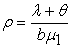and after simplification, we get(13)

where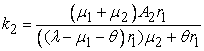and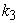= -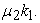Solving equation (13), ((=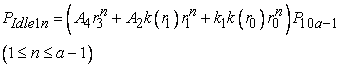(14)

here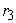=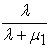and k(x) =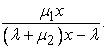Solving equation (8)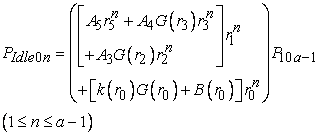(15)

here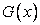=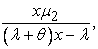=Substituting the values of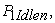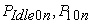andin equation (9),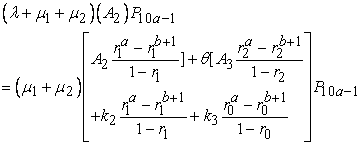(16)

After simplification, we obtain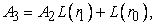(17)

whereThe value of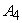can be determined using the equation (14).(18)

Also, we obtain the value of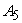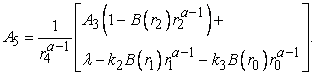(19)

The value ofis(20)

where=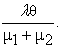Using the value ofwe can calculate the values of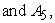All the steady state probabilities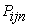for n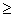0, i, j = 0,1 are obtained in terms of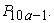The value of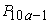can be determined using normalizing condition,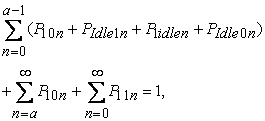(21)

we get the solution after some algebraic simplification as follows,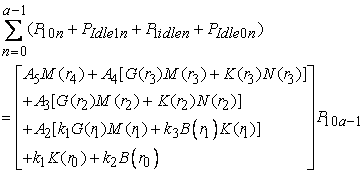(22)

Where,) =+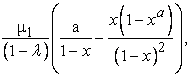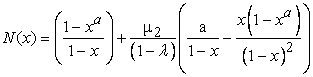then(23)

### 5. Performance Measures

The efficiency of the queueing system can be proved by finding the performance measures of the queueing systems under consideration. As the steady-state probabilities are known, various performance measures of the queue can be easily obtained.

a) Mean Queue Length

Let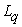be the expected number of customers in the queue then(24)

b) Probability that both the servers are busy (P2B)(25)

c) Probability that one server is busy (P1B)(26)

d) Probability that the servers either on vacation or idle (PidleB)(27)

### 6. Numerical Analysis

The numerical values of the expected queue length and various performance measures for different values of parameters a, b, λ, μ1, μ2 and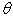which are given in the following tables

From the above Table 2 it is noted that for various values of λ andthe normalized condition total probability is approximately equal to 1. Also, when the arrival rate λ is increased, the queue length also increased.

### 7. Pictorial Representation

• Figure 1. Lq Vs λ various values of θ when a =25, b = 40, μ1=1 and μ2= 2

### 8. Conclusion

In this present study, a queueing model M/M(a,b)/(2,1) with heterogeneous servers with delayed vacation for slow server depends on batch size are considered. The two main objectives of queueing theory as the whole are to reduce the waiting time of customers and the queue length. This present study satisfies the purpose because, the fast server is always retained in the system and slow server can go only for a delayed vacation. The model proposed here is applied for many real world problems.

### References

  Borthakur, A & Choudhury, G 1997, On a batch arrival Poisson queue with generalized vacation, Sankhyā: The Indian Journal of Statistics, Series B, pp. 369-383. In article  Bruneel, H & Wuyts, I 1994, Analysis of discrete-time multi server queueing models with constant service times, Operations Research Letters, vol. 15, no. 5, pp. 231-236 In article View Article  Choudhury, G & Madan, KC 2006, A batch arrival Bernoulli vacation queue with a random setup time under restricted admissibility policy, International Journal of Operational Research, vol. 2, no. 1, pp. 81-97. In article View Article  Doshi, BT 1986, Queueing systems with vacations - a survey, Queueing systems, vol. 1, no. 1, pp. 29-66. In article View Article  Fuhrmann, S & Cooper, RB 1985, Stochastic decompositions in the M/G/1 queue with generalized vacations, Operations Research, vol. 33, no. 5, pp. 1117-1129. In article View Article  Harris, CM & Gross, D 1974, Fundamentals of Queuing theory, John Wiely & Sons. In article  Kella, O & Yechiali, U 1988, Priorities in M/G/1 Queue with Server Vacations, Naval Research Logistics, vol. 35, pp. 23-34. In article View Article  Krishnamoorthi, B 1963, On Poisson queue with two heterogeneous servers, Operations Research, vol. 11, no. 3, pp. 321-330 In article View Article  Medhi, J & Borthakur, A 1972, 'On a two server Markovian queue with a general bulk service rule', Cahiers Centre Etudes Recherch Oper, vol. 21. In article  Morse, PM 1958, Queues, inventories and maintenance, Wiley New York. In article View Article  Neuts, MF 1975, Computational uses of the method of phases in the theory of queues, Computers & Mathematics with Applications, vol. 1, no. 2, pp. 151-166 In article View Article  Singh, CJ, Jain, M & Kumar, B 2013, Analysis of queue with two phases of service and m phases of repair for server breakdown under N-policy, International Journal of Services and Operations Management, vol. 16, no. 3, pp. 373-406. In article View Article  Sree Parimala. R & Palaniammal, S 2014, Single server Queueing Model with server delayed vacation and switch over state, Applied Mathematical Sciences, vol.8, no.163, pp.8113-8124. In article View Article  Zhang, ZG & Tian, N 2004, An analysis of queueing systems with multi-task servers, European Journal of Operational Research, vol. 156, no. 2, pp. 375-389. In article View ArticleThis work is licensed under a Creative Commons Attribution 4.0 International License. To view a copy of this license, visit http://creativecommons.org/licenses/by/4.0/